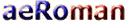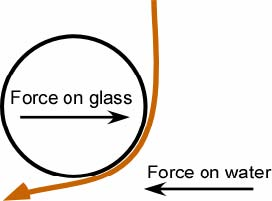As always, simple statements often result in more questions. One natural question is why does the air bend around the wing? This question is probably the most challenging question in understanding flight and it is one of the key concepts. Let us start by first looking at a simple demonstration. Run a small stream of water from a faucet and bring a horizontal water glass over to it until it just touches the water, as in Figure 9. As in the figure, the water will wrap partway around the glass. From Newton’s first law we know that for the flow of water to bend there must be a force on it. The force is in the direction of the bend. From Newton’s third law we know that there must be an equal and opposite force acting on the glass. The stream of water puts a force on the glass that tries to pull it into the stream, not push it away as one might first expect.Figure 9. Water wrapping around a glassSo why does the water bend around the glass, or air over a wing? First consider low-speed flight. In low-speed flight the forces on the air and the associated pressures are so low that the air is not only considered a fluid but an incompressible fluid. This means that the volume of a mass of air remains constant and that flows of air do not separate from each other to form voids (gaps). A second point to understand is that streamlines communicate with each other. A streamline, in steady-state flight, can be looked at as the path of a particle in the moving air. It is the path a small, light object would take in the airflow over the wing. The communication between streamlines is an expression of pressure and viscosity. Pressure is the force per area that the air exerts on the neighboring streamline. Viscosity in a gas or liquid corresponds to friction between solids. Think of two adjacent streamlines with different speeds. Since these streamlines have different velocities forces between them trying to speed up the slower streamline and slow down the faster streamline. The speed of air at the surface of the wing is exactly zero with respect to the surface of the wing. This is an expression of viscosity. The speed of the air increases with distance from the wing as shown in Figure 10. Now imagine the first non-zero velocity streamline that just grazes the highpoint of the top of the wing. If it were initially to go straight back and not follow the wing, there would be a volume of zero velocity air between it and the wing. Forces would strip this air away from the wing and without a streamline to replace it, the pressure would lower. This lowering of the pressure would bend the streamline until it followed the surface of the wing.Figure 10. The variation of the speed of a fluid near an objectThe next streamline above would be bent to follow the first by the same process, and so on. The streamlines increase in speed with distance from the wing for a short distance. This is on the order of 6 inch (15 cm) at the trailing edge of the wing of an Airbus A380. This region of rapidly changing air speed is the boundary layer. If the boundary layer is not turbulent, the flow is said to be laminar. Thus, the streamlines are bent by a lowering of the pressure. This is why the air is bent by the top of the wing and why the pressure above the wing is lowered. This lowered pressure decrease with distance above the wing but is the basis of the lift on a wing. The lowered pressure propagates out at the speed of sound, causing a great deal of air to bend around the wing. Two streamlines communicate on a molecular scale. This is an expression of the pressure and the viscosity of air. Without viscosity there would be no communication between streamlines and no boundary layer. Often, calculations of lift are made in the limit of zero viscosity. In these cases viscosity is re-introduced implicitly with the Kutta-Joukowski condition, which requires that the air come smoothly off at the trailing edge of the wing. Also, the calculations require that the air follows the surface of the wing which is another introduction of the effects of viscosity. One result of the near elimination of viscosity from the calculations is that there is no boundary layer calculated.It should be noted that the speed of the uniform flow over the top of the wing is faster then the free-stream velocity, which is the velocity of the undisturbed air some distance from the wing. The bending of the air causes the reduction in pressure above the wing. This reduction in pressure causes an acceleration of the air. It is often taught that the acceleration of the air causes a reduction in pressure. In fact, it is the reduction of pressure that accelerates the air in agreement with Newton’s first law. Let us look at the air bending around the wing in Figure 11. To bend the air requires a force. As indicated by the colored arrows, the direction of the force on the air is perpendicular to the bend in the air. The magnitude of the force is proportional to the tightness of the bend. The tighter the air bends the greater the force on it. The forces on the wing, as shown by the black arrows in the figure, have the same magnitude as the forces on the air but in the opposite direction. These forces, working through pressure, represent the mechanism in which the force is transferred to the wing.Figure 11. Forces on the air and the corresponding reaction forces on the wingLook again at Figure 11, while paying attention to the black arrows representing the forces on the wing. There are two points to notice. The first is that most of the lift is on the forward part of the wing. In fact, half of the total lift on a wing at subsonic speeds is typically produced in the first one-fourth of the chord length. The chord is the distance from the leading edge to the trailing edge of the wing. The second thing to notice is that the arrows on the leading part of the wing are tilted forward. Thus the force of lift is pulling the wing along as well as lifting it. This would be nice if it were the entire story. Unfortunately, the horizontal forces on the trailing part of the wing compensate the horizontal forces on the leading part of the wing. We now have the tools to understand why a wing has lift. In brief, the air bends around the wing producing downwash. Newton’s first law says that the bending of the air requires a force on the air, and Newton’s third law says that there is an equal and opposite force on the wing. That is a description of lift. The pressure difference across the wing is the mechanism in which lift is transferred to the wing due to the bending of the air.MORE (Lift as a Function of Angle of Attack) Source: David Anderson, Scott Eberhardt [HOME] [AVIATION] [How Airplanes fly] [History] [Records] [Humor] [News] [Photos] [Stories] [Stamps] [Sounds] [Movies] [HUMANS] [ORIGINS] [SCIENCE] [WEB LINKS] [SITE MAP] [CONTACT]